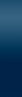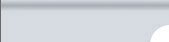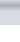MenuToolbarConstraints/ResultsImpedance SpectraEquivalent CircuitSettings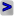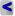EIS Spectrum Analyser Help Menu: Linear Kramers-Kronig Test Analysis / Linear KK Check. Generates a plot of residuals between imaginary (real) part of impedance of Voigt -RC- model and imaginary (real) part of impedance of experimental data. Linear Kramers-Kronig test realized in this program is based on approach developed by M.E. Orazem and co-workers and further modified by B. Boukamp in [J. Electrochem. Soc., 142 (1995) 1885]. In this approach the Kramers-Kronig transform is replaced by the fit to the Voigt -RC- model. If the data cannot be well approximated by the Voigt model containing reasonable number of -RC- pairs, it means that data are not transformable. The routine uses fit of data to the following equations: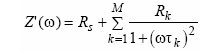and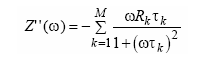Where τk are time constants (τk = RkCk) distributed logarithmically within the measured frequency range. Examination of the relative residual plot between measured and approximated spectra give information about the measured data quality. To prevent noise averaging, the number of time constants τk is somewhat smaller than number of data points. You can use different number of time constants with respect to data points by selecting Time constants/Data points ratio in Linear Kramers-Kronig test form. Click Check button in Linear Kramers-Kronig test form to restart test after changing of Time constants/Data points ratio. See also Logarithmic Kramers-Kronig test EIS Spectrum Analyser, 2008